## Monday, April 25, 2016

### Making a Sharpening Block for a Scrub Plane Iron - Resolution

Warning: this post may only be interesting to math geeks like myself.  I don't intend to to this often in my posts.  Only when I need to remember how I figured something out.

Recall that I wanted to figure out the radius of concave arc to shape a wooden block so that I can use it with sandpaper to sharpen a scrub plane iron that has an 8" radius.  If the iron was standing straight up (90° to the block), then an 8" radius on the block would be OK.Iron at 90° angle to block
But since the iron will be sharpened at a 30° angle, an 8" radius on the block would not be the correct radius.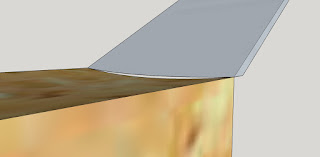An 8" radius on the block does not match the iron when the iron is at 30° to the block.  Mind the gap!

Well, this has been rumbling around my brain for a few days and I finally came up with a solution today while on my bicycle.  Consider an 8" long plane iron with 8" radius on the business end.  In the following picture, the center of an 8" radius circle is the center of the back end of the iron.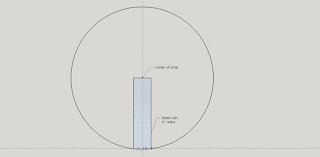Iron shown with 8" radius circle
Now draw two lines from center of the circle to the sides of the 2" wide iron at business end.  The segments are OA and OB.  Point "C" is along a straight line connecting A and B.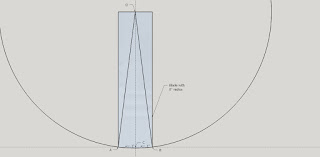Closer picture of iron with lines OA and OB drawn (didn't really need to draw OB)
Since the blade is 2" wide, the distance between A and B is 2".  Point C is midway between A and B and so the distance between A and C is 1", as is the distance between C and B.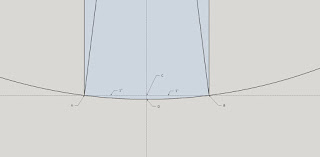Close-up of the radiused end of the iron
I want to find the distance between points C and D.  That tells me how far the center of the blade extends past the sides of the blade.  Consider the right triangle OCA.  Since the radius of the circle is 8", the length of OA is 8" and the length of OD is 8".  Therefore, the length of OC is 8" minus the length of CD.

Angle OCA is a right angle, making triangle OCA a right triangle and the sum of the squares of the legs is equal to the square of the hypotenuse.

OC^2 + AC^2 = OA^2
(8 - CD)^2 + 1^2 = 8^2
8 - CD = SQRT(63)
CD = 8 - SQRT(63) = 0.063
.
Now consider the plane blade resting at a 30° angle on a 2" wide block of wood.  Pretend the edges of the blade (points A and B) are resting on the edges of the block, so that the center of the blade is protruding into the wood.  The question is: how deep vertically is the center of the blade.

Here is a cross section of that scenario.  This is a side view of the plane iron extending into the block.  This cross section shows the centerline of the block (lengthwise).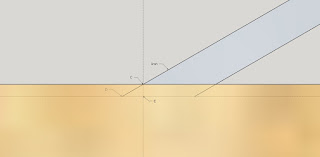Cross section of center of block and iron
Not sure if this is clear enough.  But in this picture I want to figure out distance CE, which will tell me how big of an arc to cut into the block.  Triangle CDE is another right triangle and angle CDE is 30° since the horizontal dotted line is parallel to the block surface and the vertical dotted line is perpendicular to it.

With a little trig,
sin(30°) = CE / CD
0.5 = CE / 0.063
CE = 0.0314

OK, now that I know how deep vertically (into the block) the center of the iron is, I can figure out the radius of a circle that will give me and arc to match this depth.

Here is an end view of the block and I've drawn a new circle whose radius I need to figure out.  Let's call the center of this circle O'.  I've drawn a line from O' to one edge of the block (point A).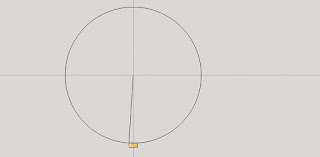Block at bottom center looking end-on
Here's a close-up of the part that matters (center of circle is out of the picture).Close-up of end-on view of block with arc from the circle that was drawn
The radius of the circle is O'A (distance from center O' to point A - the angled line in upper left goes to the center O').  This is the same as the distance from O' to E (also the radius of the circle).  Let's call that radius "z".  Since I want distance CE to be 0.0314, then O'C = z - 0.0314.

Triangle O'AC is a right triangle and by the Pythagorean theorem,

AC^2 + O'C^2 = O'A^2
1^2 + (z-0.0314)^2 = z^2
z^2 - 0.0628z + 0.0314^2 = z^2 - 1
z = 15.96 (I skipped a couple steps)

So call the radius that I need on the block 16".  With a concave arc having a radius of 16" scooped out of my block, I should be able to sharpen the blade whose edge has an 8" radius camber, when sharpening at 30° bevel angle.

As they used to say in geometry class: QED.

We now return you to our regular programming ...

1.Where is the bottle of aspirin?

2.Huhhh? But of course!
Pass the bottle Ralph :-)

3.Can we change the bottle to something stronger?

4.Oh, come one guys. It wasn't that bad, was it? At least it made me work out the brain cells a little.

5.A simple method for calculating your distance from the arc to the chord (distance C i think you labeled it) is with the formula C/2*tan(I/4). where C is the width of the blade in this problem and I is the angle between line AO and BO. Divide this number by 2 to get your distance at 30 degrees.

1.Ken, did you derive that formula or was it in a reference book somewhere?